Contemporary Mathematics

# 10.7Volume and Surface Area

Contemporary Mathematics10.7 Volume and Surface Area

Figure 10.123 Volume is illustrated in this 3-dimensional view of an interior space. This gives a buyer a more realistic interpretation of space. (credit: "beam render 10 with sun and cat tree" by monkeywing/Flickr, CC BY 2.0)

### Learning Objectives

After completing this section, you should be able to:

1. Calculate the surface area of right prisms and cylinders.
2. Calculate the volume of right prisms and cylinders.
3. Solve application problems involving surface area and volume.

Volume and surface area are two measurements that are part of our daily lives. We use volume every day, even though we do not focus on it. When you purchase groceries, volume is the key to pricing. Judging how much paint to buy or how many square feet of siding to purchase is based on surface area. The list goes on. An example is a three-dimensional rendering of a floor plan. These types of drawings make building layouts far easier to understand for the client. It allows the viewer a realistic idea of the product at completion; you can see the natural space, the volume of the rooms. This section gives you practical information you will use consistently. You may not remember every formula, but you will remember the concepts, and you will know where to go should you want to calculate volume or surface area in the future.

We will concentrate on a few particular types of three-dimensional objects: right prisms and right cylinders. The adjective “right” refers to objects such that the sides form a right angle with the base. We will look at right rectangular prisms, right triangular prisms, right hexagonal prisms, right octagonal prisms, and right cylinders. Although, the principles learned here apply to all right prisms.

### Three-Dimensional Objects

In geometry, three-dimensional objects are called geometric solids. Surface area refers to the flat surfaces that surround the solid and is measured in square units. Volume refers to the space inside the solid and is measured in cubic units. Imagine that you have a square flat surface with width and length. Adding the third dimension adds depth or height, depending on your viewpoint, and now you have a box. One way to view this concept is in the Cartesian coordinate three-dimensional space. The $xx$-axis and the $yy$-axis are, as you would expect, two dimensions and suitable for plotting two-dimensional graphs and shapes. Adding the $zz$-axis, which shoots through the origin perpendicular to the $xyxy$-plane, and we have a third dimension. See Figure 10.124.

Figure 10.124 Three-Dimensional Space

Here is another view taking the two-dimensional square to a third dimension. See Figure 10.125.

Figure 10.125 Going from Two Dimensions to Three Dimensions

To study objects in three dimensions, we need to consider the formulas for surface area and volume. For example, suppose you have a box (Figure 10.126) with a hinged lid that you want to use for keeping photos, and you want to cover the box with a decorative paper. You would need to find the surface area to calculate how much paper is needed. Suppose you need to know how much water will fill your swimming pool. In that case, you would need to calculate the volume of the pool. These are just a couple of examples of why these concepts should be understood, and familiarity with the formulas will allow you to make use of these ideas as related to right prisms and right cylinders.

Figure 10.126

### Right Prisms

A right prism is a particular type of three-dimensional object. It has a polygon-shaped base and a congruent, regular polygon-shaped top, which are connected by the height of its lateral sides, as shown in Figure 10.127. The lateral sides form a right angle with the base and the top. There are rectangular prisms, hexagonal prisms, octagonal prisms, triangular prisms, and so on.

Figure 10.127 Pentagonal Prism

Generally, to calculate surface area, we find the area of each side of the object and add the areas together. To calculate volume, we calculate the space inside the solid bounded by its sides.

### FORMULA

The formula for the surface area of a right prism is equal to twice the area of the base plus the perimeter of the base times the height, $SA=2B+ph,SA=2B+ph,$ where $BB$ is equal to the area of the base and top, $pp$ is the perimeter of the base, and $hh$ is the height.

### FORMULA

The formula for the volume of a rectangular prism, given in cubic units, is equal to the area of the base times the height, $V=B⋅h,V=B⋅h,$ where $BB$ is the area of the base and $hh$ is the height.

### Example 10.56

#### Calculating Surface Area and Volume of a Rectangular Prism

Find the surface area and volume of the rectangular prism that has a width of 10 cm, a length of 5 cm, and a height of 3 cm (Figure 10.128).

Figure 10.128

1.
A rectangular solid has a width of 6 cm, length of 15 cm, and height or depth of 6 cm. Find the surface area and the volume.

In Figure 10.129, we have three views of a right hexagonal prism. The regular hexagon is the base and top, and the lateral faces are the rectangular regions perpendicular to the base. We call it a right prism because the angle formed by the lateral sides to the base is $90∘.90∘.$ See Figure 10.127.

Figure 10.129 Right Hexagonal Prism

The first image is a view of the figure straight on with no rotation in any direction. The middle figure is the base or the top. The last figure shows you the solid in three dimensions. To calculate the surface area of the right prism shown in Figure 10.129, we first determine the area of the hexagonal base and multiply that by 2, and then add the perimeter of the base times the height. Recall the area of a regular polygon is given as $A=12ap,A=12ap,$ where $aa$ is the apothem and $pp$ is the perimeter. We have that

$Abase=12(5.2)(36)=93.6cm2Abase=12(5.2)(36)=93.6cm2$

Then, the surface area of the hexagonal prism is

$SA=2(93.6)+36(20)=907.2in2SA=2(93.6)+36(20)=907.2in2$

To find the volume of the right hexagonal prism, we multiply the area of the base by the height using the formula $V=Bh.V=Bh.$ The base is $93.6cm2,93.6cm2,$ and the height is 20 $cmcm$. Thus,

$V=93.6(20)=1872cm3.V=93.6(20)=1872cm3.$

### Example 10.57

#### Calculating the Surface Area of a Right Triangular Prism

Find the surface area of the triangular prism (Figure 10.130).

Figure 10.130

1.
Find the surface area of the triangular prism shown.

### Example 10.58

#### Finding the Surface Area and Volume

Find the surface area and the volume of the right triangular prism with an equilateral triangle as the base and height (Figure 10.131).

Figure 10.131

1.
Find the surface area and the volume of the octagonal figure shown.

### Example 10.59

#### Determining Surface Area Application

Katherine and Romano built a greenhouse made of glass with a metal roof (Figure 10.132). In order to determine the heating and cooling requirements, the surface area must be known. Calculate the total surface area of the greenhouse.

Figure 10.132

1.
Calculate the surface area of a greenhouse with a flat roof measuring 12 ft wide, 25 ft long, and 8 ft high.

### Right Cylinders

There are similarities between a prism and a cylinder. While a prism has parallel congruent polygons as the top and the base, a right cylinder is a three-dimensional object with congruent circles as the top and the base. The lateral sides of a right prism make a $90∘90∘$ angle with the polygonal base, and the side of a cylinder, which unwraps as a rectangle, makes a $90∘90∘$ angle with the circular base.

Right cylinders are very common in everyday life. Think about soup cans, juice cans, soft drink cans, pipes, air hoses, and the list goes on.

In Figure 10.133, imagine that the cylinder is cut down the 12-inch side and rolled out. We can see that the cylinder side when flat forms a rectangle. The $SASA$ formula includes the area of the circular base, the circular top, and the area of the rectangular side. The length of the rectangular side is the circumference of the circular base. Thus, we have the formula for total surface area of a right cylinder.

Figure 10.133 Right Cylinder

### FORMULA

The surface area of a right cylinder is given as $SA=2πr2+2πrh.SA=2πr2+2πrh.$

To find the volume of the cylinder, we multiply the area of the base with the height.

### FORMULA

The volume of a right cylinder is given as $V=πr2h.V=πr2h.$

### Example 10.60

#### Finding the Surface Area and Volume of a Cylinder

Given the cylinder in Figure 10.133, which has a radius of 5 inches and a height of 12 inches, find the surface area and the volume.

1.
Find the surface area and volume of the cylinder with a radius of 7cm and a height of 5 cm.

### Applications of Surface Area and Volume

The following are just a small handful of the types of applications in which surface area and volume are critical factors. Give this a little thought and you will realize many more practical uses for these procedures.

### Example 10.61

#### Applying a Calculation of Volume

A can of apple pie filling has a radius of 4 cm and a height of 10 cm. How many cans are needed to fill a pie pan (Figure 10.134) measuring 22 cm in diameter and 3 cm deep?

Figure 10.134

1.
You are making a casserole that includes vegetable soup and pasta. The size of your cylindrical casserole dish has a diameter of 10 inches and is 4 inches high. The pasta will consume the bottom portion of the casserole dish about 1 inch high. The soup can has a diameter of 3 inches and is 4 inches high. After the pasta is added, how many cans of soup can you add?

### Optimization

Problems that involve optimization are ones that look for the best solution to a situation under some given conditions. Generally, one looks to calculus to solve these problems. However, many geometric applications can be solved with the tools learned in this section. Suppose you want to make some throw pillows for your sofa, but you have a limited amount of fabric. You want to make the largest pillows you can from the fabric you have, so you would need to figure out the dimensions of the pillows that will fit these criteria. Another situation might be that you want to fence off an area in your backyard for a garden. You have a limited amount of fencing available, but you would like the garden to be as large as possible. How would you determine the shape and size of the garden? Perhaps you are looking for maximum volume or minimum surface area. Minimum cost is also a popular application of optimization. Let’s explore a few examples.

### Example 10.62

#### Maximizing Area

Suppose you have 150 meters of fencing that you plan to use for the enclosure of a corral on a ranch. What shape would give the greatest possible area?

1.
You have 25 ft of rope to section off a rectangular-shaped garden. What dimensions give the maximum area that can be roped off?

Suppose you want to design a crate built out of wood in the shape of a rectangular prism (Figure 10.136). It must have a volume of 3 cubic meters. The cost of wood is $15 per square meter. What dimensions give the most economical design while providing the necessary volume? Figure 10.136 ### Your Turn 10.63 1. Suppose you want to a build a container to hold 2 cubic feet of fabric swatches. You want to cover the container in laminate costing$10 per square foot. What are the dimensions of the container that is the most economical? What is the cost?

42.
Find the surface area of the equilateral triangular prism shown.43.
Find the surface area of the octagonal prism shown.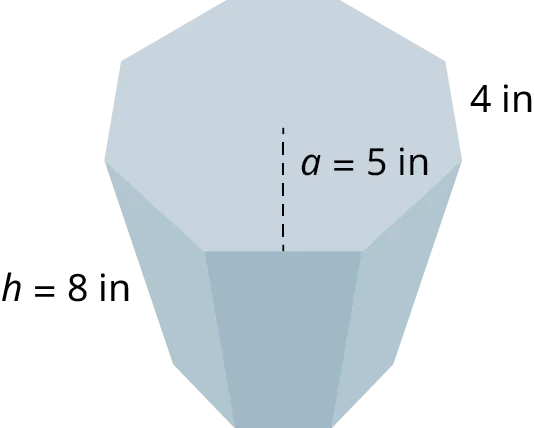44.
Find the volume of the octagonal prism shown with the apothem equal to $5\,\text{in},$ the side length equal to $4\,\text{in},$ and the height equal to $8\,\text{in}.$
45.
Determine the surface area of the right cylinder where the radius of the base is $10\,\text{cm}$, and the height is $5\,\text{cm}$.
46.
Find the volume of the cylinder where the radius of the base is $10\,\text{cm}$, and the height is $5\,\text{cm}$.
47.
As an artist, you want to design a cylindrical container for your colored art pencils and another rectangular container for some other tools. The cylindrical container will be 8 inches high with a diameter of 6 inches. The rectangular container measures 10 inches wide by 8 inches deep by 4 inches high and has a lid. You found some beautiful patterned paper to use to wrap both pieces. How much paper will you need?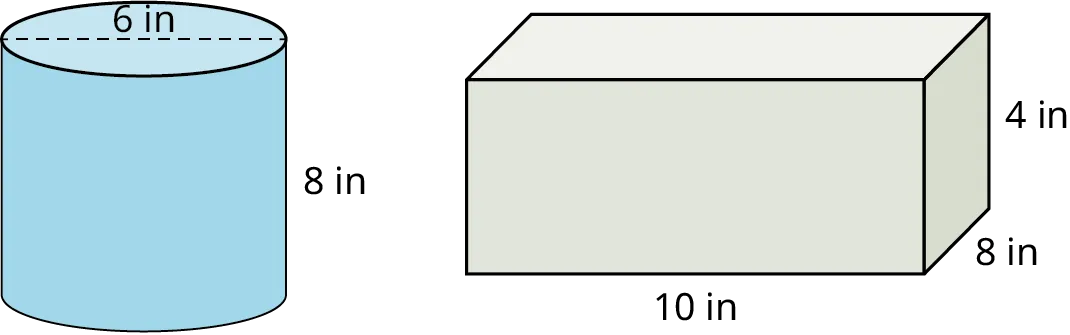### Section 10.7 Exercises

1 .
Find the volume of the right triangular prism with the two side legs of the base equal to 10 m, the hypotenuse equal to $10\sqrt 2$, and the height or the length, depending on your viewpoint, is equal to 15 m.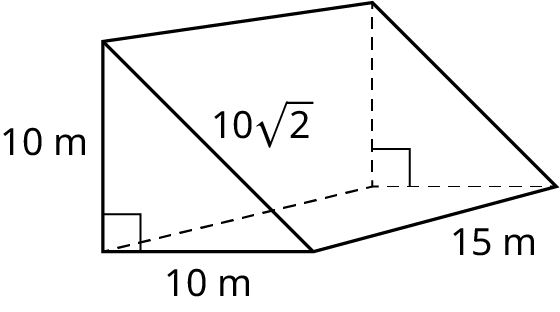2 .
Find the surface area of the right triangular prism in the Exercise 1 with the two legs of the base equal to 10 m, and the height equal to 15 m.
3 .
Find the surface area of the right trapezoidal prism with side ${\text{a = }}5\,{\text{cm}}$, side ${\text{b = }}{\text{12}}\,{\text{cm}}$, the height is 10 cm, the slant length is 12 cm, and the length is 24 cm.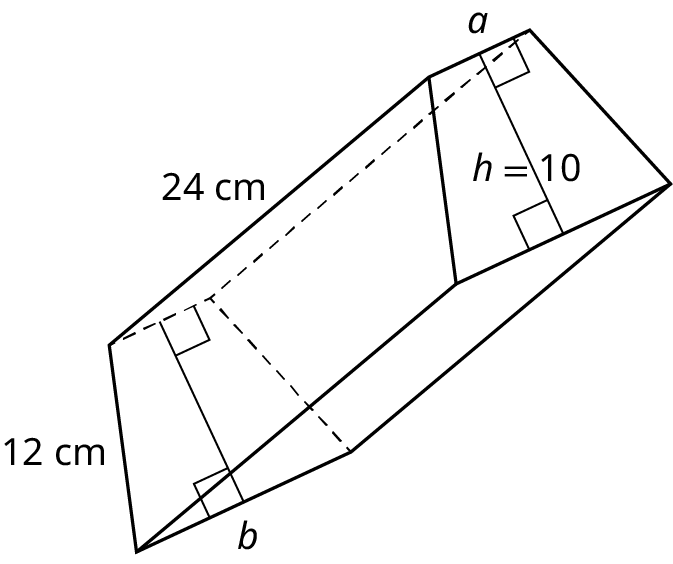4 .
Find the volume of the trapezoidal prism in the exercise above where the base and top have the following measurements: side $a = 5\,\text{cm}$, side $b = 12\,\text{cm}$, the slant lengths are each $12\,\text{cm}$, and the height of the trapezoidal base =$10\,\text{cm}$. The height or length of the three-dimensional solid is $24\,\text{cm}$.
5 .
Find the surface area of the octagonal prism. The base and top are regular octagons with the apothem equal to 10 m, a side length equal to 12 m, and a height of 30 m.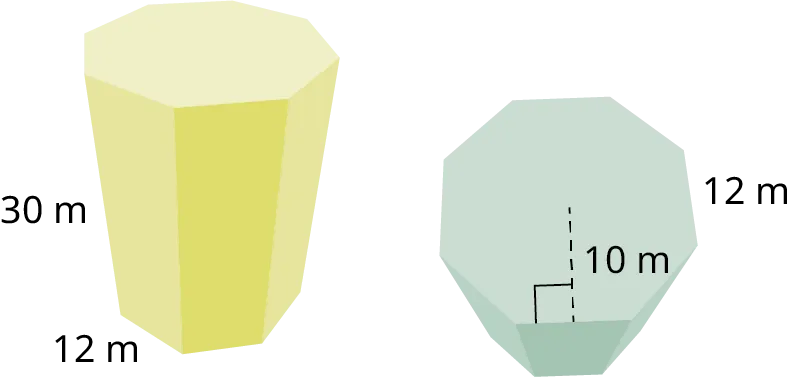6 .
Find the volume for the right octagonal prism, with the apothem equal to 10 m, the side length of the base is equal to 12 m, and the height equal to 30 m.
7 .
You decide to paint the living room. You will need the surface area of the 4 walls and the ceiling. The room measures 20 ft long and 14 ft wide, and the ceiling is 8 ft high.
For the following exercises, find the surface area of each right cylinder.
8 .
$r = 6\,\text{cm},\,h = 5\,\text{cm}$
9 .
$r = 9\,\text{cm},\,h = 15\,\text{cm}$
10 .
$r = 12.2\,\text{in},\,h = 30\,\text{in}$
11 .
$r = 3.4\,\text{m},\,h = 10.5\,\text{m}$
12 .
$d = 11.4\,\text{m},\,h = 4.4\,\text{m}$
13 .
$d = 20\,\text{in},\,h = 8.5\,\text{in}$
For the following exercises, find the volume of each right cylinder to the nearest tenth.
14 .
$r = 7\,\text{cm},\,h = 8\,\text{cm}$
15 .
$r = 14\,\text{cm},\,h = 19\,\text{cm}$
16 .
$r = 14\,\text{in},\,h = 30\,\text{in}$
17 .
$r = 4.9\,\text{m},\,h = 17\,\text{m}$
18 .
$r = 12.6\,\text{cm},\,h = 16.2\,\text{cm}$
19 .
You have remodeled your kitchen and the exhaust pipe above the stove must pass through an overhead cabinet as shown in the figure. Find the volume of the remaining space in the cabinet.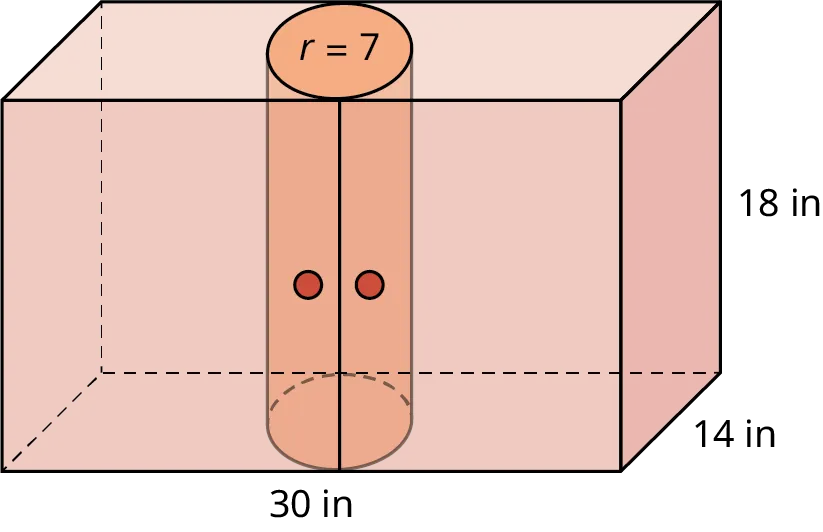Order a print copy

As an Amazon Associate we earn from qualifying purchases.